# 分支控制

if语句 语法如下:
if(表达式){

...
}

if else语句。语法如下
if(表达式){

...
}else{

...
}

$age=19; if ($age>18){
echo '你已经成年啦';             #因为$age=19 大于18，所以表达式为真，所以输出后面得。所以输出“你已经成年啦” }else{ echo '小可爱，你还未成年！'; }  多分支控制 语法： if(){ 语句1； ... }else if(){ 语句1； ... }else{ 语句1； ... } 这里得else if(){}可以加很多个。 举个例子，比如： $age=3;
if ($age>18){ echo '你已经成年啦'; }elseif($age>10 && $age<18){ echo '是少年啦'; }elseif($age>5 && $age<10){ echo '你还是个孩子'; }else{ echo '是婴儿啦'; }  多分支还有switch语句。语法如下。 switch(表达式){ case常量1: break; case常量2: break; case常量3: break; case常量4: break; ... } 举个例子： $a=1;
switch ($a){ case 1: echo '今天星期1'; break; case 2: echo '今天星期2'; break; case 3: echo '今天星期3'; break; //这里可以写很多个 default: echo '输入有误'; }  这里需要注意得是，case语句得break不可以省略，否则无法跳出switch语句，继续向下执行，直到遇到break。还有一点需要注意得是，default语句放在哪里没影响，程序执行顺序是，先查找case语句，case语句没有符合得，在寻找default语句。 区分一下，什么时候用if语句，什么时候用switch语句。 当控制得是一个范围时，一般用if语句，当控制得是每个点时，用switch语句。 # 循环控制 for循环控制。 语法为for(循环初值；循环得条件；步长){ 语句..... } 举个例子： for ($age=0;$age<20;$age++){
echo '

}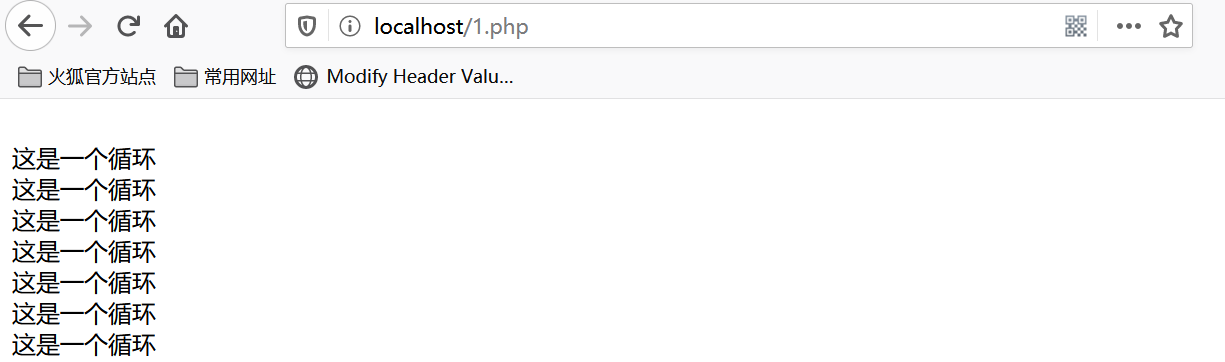while循环

while(循环控制){

}

$a=0; while ($a<10){

echo '<br/>这是while循环'.$a;$a++;
}
#循环语句中一定要有控制语句，在这里是$a++，如果不加，0永远小于10，这个一个死循环。  do while循环 循环格式为： do{ 循环语句 }while(循环控制); 举个例子： $i=0;
do{
echo '<br/>这是一个do while循环'.$i;$i++;
}while($i<10); 这里也一样，如果不加$i++控制，也会成为死循环。


# 实例

## switch分支完成简单计算器。

<html>
<title>这是一个计算器</title>
<body >
#由于是本地，所以将表单提交到okok.php。
<form action="okok.php" method="post">
<table>
<tr><td>请输入</td><td><input type="text" name="sum1"></td></tr><br/>
<tr><td>请在输入</td><td><input type="text" name="sum2"></td></tr>
<tr><td>点击计算</td><td><input type="submit"></td></tr>
</table>
<select name="oper" >
<option value="+">+</option>
<option value="-">-</option>
<option value="*">*</option>
<option value="/">/</option>
</select>
</form>
</body>
</html>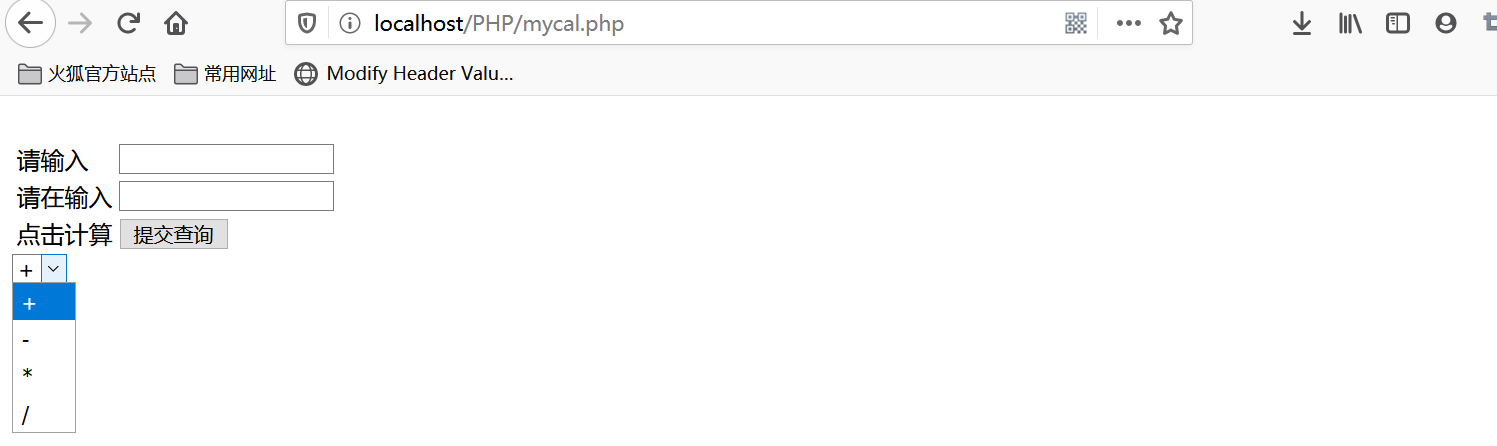<?php
$sum1=$_POST['sum1'];
$sum2=$_POST['sum2'];
$oper=$_POST['oper'];
$res=0; switch ($oper){
case "+":
$res=$sum1+$sum2; break; case "-":$res=$sum1-$sum2;
break;
case "*":
$res=$sum1*$sum2; break; case "/":$res=$sum1/$sum2;
break;
default:
echo '运算符不合法';
}
echo '运算结果为:'.$res.'<br/>'; ?> <a href="mycal.php">返回初页面</a>  现在来运算一下，输入123+456，查看返回结果。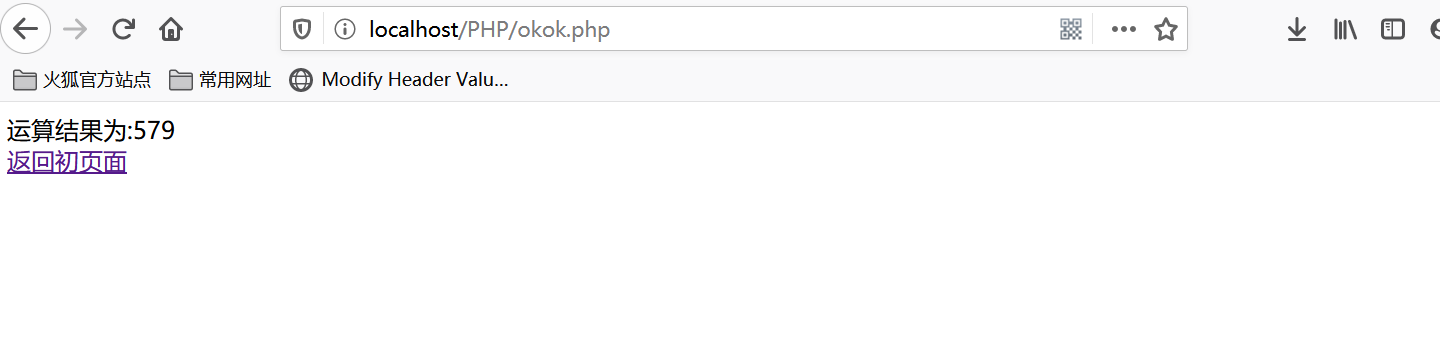## for循环打印金字塔 <?php #第一层for循环控制金字塔的层数 for ($i=1;$i<=5;$i++){
#控制"*"号
for ($j=1;$j<=$i;$j++){
echo '*';
}
echo '<br/>';
}
?>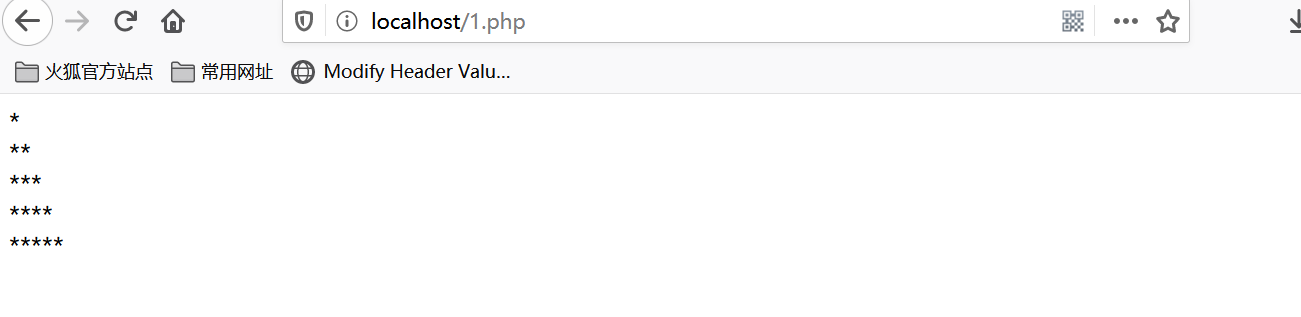<?php
#第一层for循环控制金字塔的层数
for ($i=1;$i<=5;$i++){ #控制空格 for ($k=1;$k<=5-$i;$k++){ echo '&nbsp;'; } #控制"*"号 for ($j=1;$j<=2*$i-1;$j++){ echo '*'; } echo '<br/>'; } ?>  我们再进阶一下，如果将这个金字塔内部掏空，又该如何实现呢？我们想一下，是不是我们要只要控制好两个边缘的"*"号，其他地方控制填充空格就可以了，有了思路，我们看代码，又补充了哪些地方。看代码： <?php #第一层for循环控制金字塔的层数 for ($i=1;$i<=5;$i++){
#控制空格
for ($k=1;$k<=5-$i;$k++){
echo '&nbsp;';
}
#控制"*"号
for ($j=1;$j<=2*$i-1;$j++){
if ($j==1 ||$j==2*$i-1){ echo '*'; }else{ #填充空格 echo '&nbsp;'; } } echo '<br/>'; } ?>  效果如下图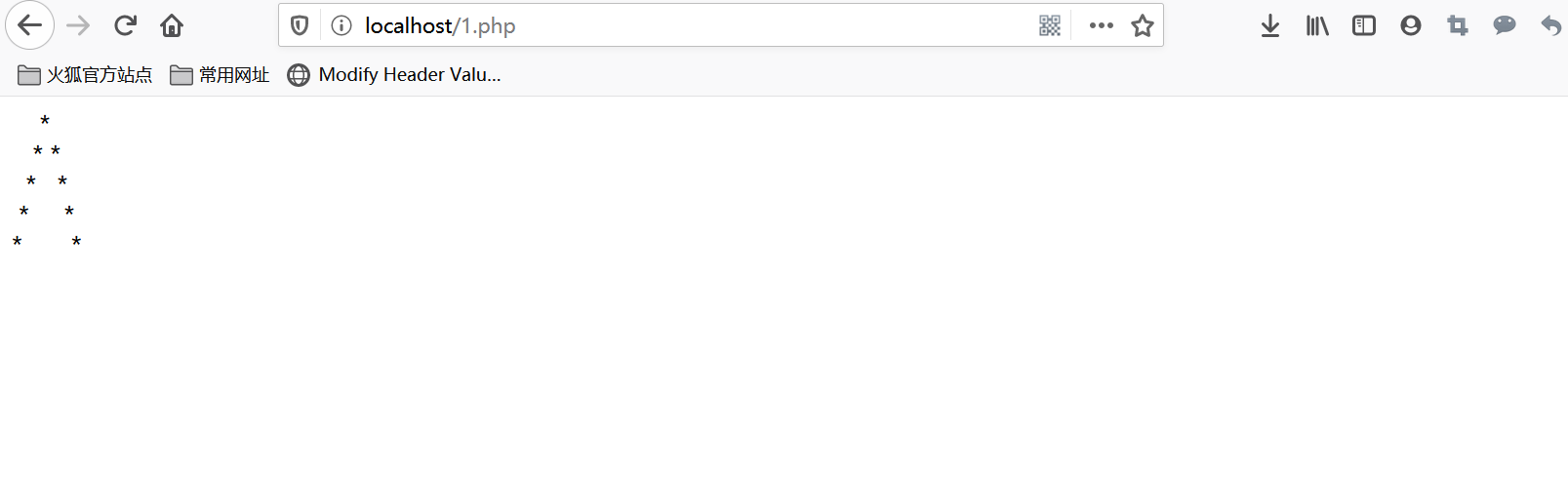咱们再进阶一下，如果将这个空心的三角翻转一下，打印出菱形，那么又该如何实现呢？是不是再原来的基础上再控制一下层数、"*"号、空格就可以完成呢。现在咱们看代码。 <?php #第一层for循环控制金字塔的层数 for ($i=1;$i<=5;$i++) {
#控制空格
for ($k = 1;$k <= 5 - $i;$k++) {
echo '&nbsp;';
}
#控制"*"号
for ($j = 1;$j <= 2 * $i - 1;$j++) {
if ($j == 1 ||$j == 2 * $i - 1) { echo '*'; } else { #填充空格 echo '&nbsp;'; } } echo '<br/>'; } #翻转 for ($i=5;$i>=0;$i--){
#控制空格
for ($k=0;$k<=5-$i;$k++){
echo '&nbsp;';
}
#控制*和空格
for ($j=1;$j<=2*$i-3;$j++){
if ($j==1 ||$j==2*\$i-3){
echo '*';
}else{
echo '&nbsp;';
}
}
echo '<br/>';
}
?>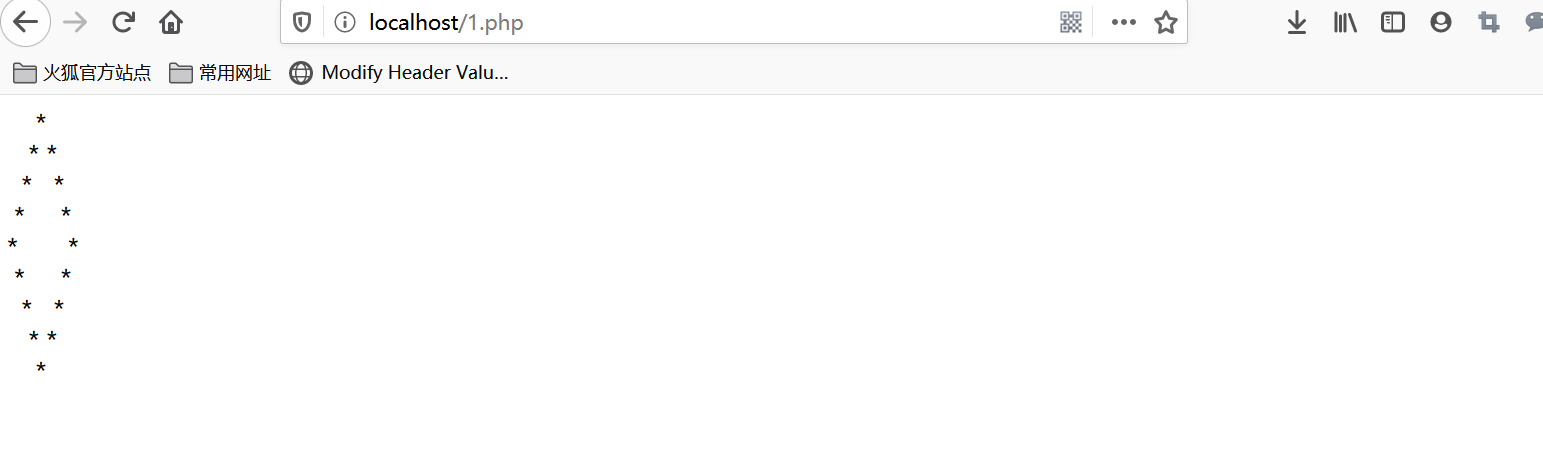# 小结

posted @ 2020-07-26 14:03  雪痕*  阅读(168)  评论(2编辑  收藏  举报